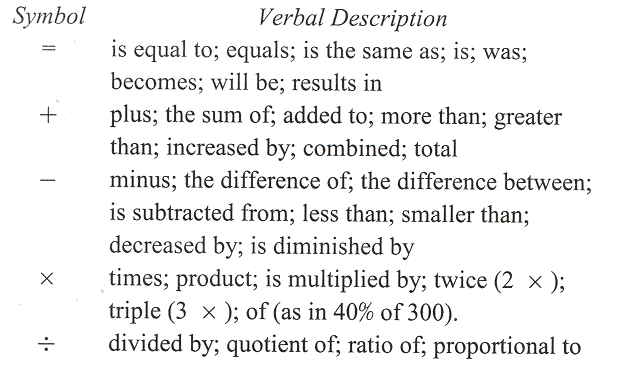# Section 6.6: Algebra in Problem Solving

## 6.6 Outline

1. Introduction to problem solving
1. principle of substitution
2. procedure
3. translating
1. sum
2. difference
3. product
4. quotient
2. Number relationships
1. formulate an equation
2. consecutive integers
3. Distance relationships
4. Pythagorean relationships
1. If two sides of a right triangle are know, the third can be found by using the Pythagorean theorem.
2. If a right triangle has sides a and b and hypotenuse c, then a2 + b2 = c2.

## 6.6 Essential Ideas

PROCEDURE FOR PROBLEM SOLVING IN ALGEBRA
Step 1: Understand the problem. This means you must read the problem and note what it is about. Focus on processes rather than numbers. You cannot work a problem you do not understand. A sketch may help in understanding the problem.
Step 2: Devise a plan. Write down a verbal description of the problem using operation signs and an equal or inequality sign. Note the following common translations.Step 3: Carry out the plan. In the context of word problems, we need to proceed deductively by carrying out the following steps.
Choose a variable. If there is a single unknown, choose a variable. If there are several unknowns, you can use the substitution property to reduce the number of unknowns to a single variable. Later we will consider word problems with more than one unknown.
Substitute. Replace the verbal phrase for the unknown with the variable.
Solve the equation. This is generally the easiest step. Translate the symbolic statement (such as x = 3) into a verbal statement. Probably no variables were given as part of the word problem, so x = 3 is not an answer. Generally, word problems require an answer stated in words. Pay attention to units of measure and other details of the problem.
Step 4: Look back. Be sure your answer makes sense by checking it with the original question in the problem. Remember to answer the question that was asked.